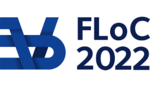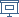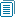FLOC 2022: FEDERATED LOGIC CONFERENCE 2022
LICS ON FRIDAY, AUGUST 5TH
Days:
previous day
all days

View: session overviewtalk overviewside by side with other conferences

08:30-09:00Coffee & Refreshments
09:00-10:00 Session 73E: Invited talk by Mikolaj Bojanczyk

Transducers of polynomial growth

Abstract: The polyregular functions are a class of string-to-string functions that have polynomial size outputs, and which can be defined using finite state models. There are many equivalent definitions of this class, with roots in automata theory, programming  languages and logic. The talk surveys recent results on polyregular functions. It presents five of the equivalent definitions, and gives self-contained proofs for most of the equivalences. Decision problems as well as restricted subclasses of the polyregular functions are also discussed.

Location: Taub 1
10:00-10:30 Session 75: Poster Session

Daniel Gratzer. Normalization for multimodal type theory

Clemens Grabmayer. Milner's Proof System for Regular Expressions Modulo Bisimilarity is Complete

Soumodev Mal. On the Satisfiability of Context-free String Constraints with Subword Ordering

Matthias Naaf. Zero-One Laws and Almost Sure Valuations of First-Order Logic in Semiring Semantics

Jana Hofmann. Deciding Hyperproperties combined with Functional Specifications

10:30-11:00Coffee Break
11:00-12:30 Session 76G: Verification

"Verification": 6 papers (12 min presentation + 2-3 min Q&A)

Location: Taub 1
11:00
On the Satisfiability of Context-free String Constraints with Subword OrderingPRESENTER: Soumodev Mal

ABSTRACT. We consider a variant of string constraints given by membership constraints in context-free languages and subword relation between variables. The satisfiability problem for this variant turns out to be undecidable. We consider a fragment in which the subword order constraints do not impose any cyclic dependency between variables. We show that this fragment is NEXPTIME-complete. As an application of our result, we settle the complexity of control state reachability in acyclic lossy channel pushdown systems, an important distributed system model. The problem was shown to be decidable by Atig et al. in 2008. However, no elementary upper bound was known. We show that this problem is NEXPTIME-complete.

11:15
The complexity of soundness in workflow nets

ABSTRACT. Workflow nets are a popular variant of Petri nets that allow for the algorithmic formal analysis of business processes. The central decision problems concerning workflow nets deal with soundness, where the initial and final configurations are specified. Intuitively, soundness states that from every reachable configuration one can reach the final configuration. We settle the widely open complexity of the three main variants of soundness: classical, structural and generalised soundness. The first two are EXPSPACE-complete, and, surprisingly, the latter is PSPACE-complete, thus computationally simpler.

11:30
Lower Bounds for the Reachability Problem in Fixed Dimensional VASSesABSTRACT. We study the complexity of the reachability problem for Vector Addition Systems with States (VASSes) in fixed dimensions. We provide four lower bounds improving the currently known state-of-art: 1) NP-hardness for unary flat 4-VASSes (VASSes in dimension 4), 2) PSpace-hardness for unary 5-VASSes, 3) ExpSpace-hardness for binary 6-VASSes and 4) Tower-hardness for unary 8-VASSes.

11:45
Probabilistic Verification Beyond Context-FreenessPRESENTER: Andrzej Murawski

ABSTRACT. Probabilistic pushdown automata (recursive state machines) are a widely known model of probabilistic computation associated with many decidable problems about termination (time) and linear-time model checking. Higher-order recursion schemes (HORS) are a prominent formalism for the analysis of higher-order computation. Recent studies showed that, for the probabilistic variant of HORS, even the basic problem of determining whether a scheme terminates almost surely is undecidable. Moreover, the undecidability already holds for order-2 schemes (order-1 schemes are known to correspond to pushdown automata). Motivated by these results, we study restricted probabilistic tree-stack automata (rPTSA), which in the nondeterministic setting are known to characterise a proper extension of context-free languages, namely, the multiple context-free languages. We show that several verification problems, such as almost-sure termination, positive almost-sure termination and omega-regular model checking are decidable for this class. At the level of higher-order recursion schemes, this corresponds to being able to verify a probabilistic version of the so-called affine-additive higher-order recursion schemes (PAHORS). PAHORS extend order-1 recursion schemes and are incomparable with order-2 schemes.

12:00
Ramsey Quantifiers over Automatic Structures: Complexity and Applications to Verification

ABSTRACT. Automatic structures are infinite structures that are finitely represented by synchronized finite-state automata. We investigate Ramsey quantifiers over automatic structures, which express the existence of infinite cliques in an edge relation, and observe connections to two problems in verification: (1) reachability with (generalized) Büchi conditions in regular model checking can be seen as Ramsey quantification over transitive automatic graphs, (2) checking monadic decomposability of automatic relations can be viewed as Ramsey quantification over co-transitive automatic graphs. We provide a comprehensive complexity landscape of Ramsey quantifiers, all between NL and EXP, which yields a wealth of new results with precise complexity.

12:15
The complexity of bidirected reachability in valence systems
PRESENTER: Georg Zetzsche

ABSTRACT. An infinite-state system is bidirected (sometimes called reversible) if for every transition, there exists another with opposite effect. We study reachability in bidirected systems in the framework of valence systems, an abstract model featuring finitely many control states and an infinite-state storage that is specified by a finite graph. Picking suitable graphs yields counters as in vector addition systems, pushdowns, integer counters, and combinations. We provide a comprehensive complexity analysis. For example, the complexity of bidirected reachability drops substantially (often to polynomial time) from that of general reachability, for almost every storage mechanism where general reachability is known to be decidable.

12:30-14:00Lunch Break

Lunch will be held in Taub lobby (CP, LICS, ICLP) and in The Grand Water Research Institute (KR, FSCD, SAT).

14:00-15:30 Session 78D: Complexity and Circuits

"Complexity and Circuits": 6 papers (12 min presentation + 2-3 min Q&A)

Location: Taub 1
14:00
Separating LREC from LFP

ABSTRACT. LREC= is an extension of first-order logic with a logarithmic recursion operator. It was introduced by Grohe et al. and shown to capture the complexity class L (log-space) over trees and interval graphs. It does not capture L in general as it is contained in FPC - fixed-point logic with counting. We show that this containment is strict. In particular, we show that the path systems problem, a classic P-complete problem which is definable in LFP - fixed-point logic - is not definable in LREC. This shows that the logarithmic recursion mechanism is provably weaker than general least fixed points.

14:15
Reasonable Space for the Lambda-Calculus, Logarithmically
PRESENTER: Gabriele Vanoni

ABSTRACT. Can the lambda-calculus be considered as a reasonable computational model? Can we use it for measuring the time and space consumption of algorithms? While the literature contains positive answers about time, much less is known about space. This paper presents a new reasonable space cost model for the lambda-calculus, given by the space used by a variant over the Krivine abstract machine, and which is the first one able to account for logarithmic space. Moreover, we study the time behavior of our machine and show how to transport the results to the call-by-value lambda-calculus.

14:30
PRESENTER: Gianluca Curzi

ABSTRACT. Circular (or cyclic) proofs have received increasing attention in recent years, and have been proposed as an alternative setting for studying (co)inductive reasoning. In particular, now several type systems based on circular reasoning have been proposed. However, little is known about the complexity theoretic aspects of circular proofs, which exhibit sophisticated loop structures atypical of more common 'recursion schemes'.

This paper attempts to bridge the gap between circular proofs and implicit computational complexity (ICC). Namely we introduce a circular proof system based on Bellantoni and Cook's famous safe-normal function algebra, and we identify proof theoretical constraints, inspired by ICC, to characterise the polynomial-time and elementary computable functions. Along the way we introduce new recursion theoretic implicit characterisations of these classes that may be of interest in their own right.

14:45
PRESENTER: Klara Nosan

ABSTRACT. We study the Radical Identity Testing problem (RIT): Given an algebraic circuit representing a multivariate polynomial $f(x_1, \dots, x_k)$ and nonnegative integers $a_1, \dots, a_k$ and $d_1, \dots, d_k$, written in binary, test whether the polynomial vanishes at the real radicals $\sqrt[d_1]{a_1}, \dots,\sqrt[d_k]{a_k}$, i.e., test whether $f(\sqrt[d_1]{a_1}, \dots, \sqrt[d_k]{a_k}) = 0$. We place the problem in coNP assuming the Generalised Riemann Hypothesis (GRH), improving on the straightforward PSPACE upper bound obtained by reduction to the existential theory of reals. Next we consider a restricted version, called 2-RIT, where the radicals are square roots of prime numbers, written in binary. It was known since the work of Chen and Kao that 2-RIT is at least as hard as the polynomial identity testing problem, however no better upper bound than PSPACE was known prior to our work. We show that 2-RIT is in coRP assuming GRH and in coNP unconditionally. Our proof relies on theorems from algebraic and analytic number theory, such as the Chebotarev density theorem and quadratic reciprocity.

15:00
Complexity of Modular Circuits
PRESENTER: Piotr Kawałek

ABSTRACT. We study how the complexity of modular circuits computing AND depends on the depth of the circuits and the prime factorization of the modulus they use. In particular, our construction of subexponential circuits of depth 2 for AND helps us to classify (modulo Exponential Time Hypothesis) modular circuits with respect to the complexity of their satisfiability. We also study a precise correlation between this complexity and the sizes of modular circuits realizing AND. On the other hand showing that AND can be computed by a polynomial size probabilistic modular circuit of depth 2 (with O(log n) random bits) providing a probabilistic computational model that can not be derandomized.

We apply our methods to determine (modulo ETH) the complexity of solving equations over groups of symmetries of regular polygons with an odd number of sides. These groups form a paradigm for some of the remaining cases in characterizing finite groups with respect to the complexity of their equation solving.

15:15
Choiceless Polynomial Time with Witnessed Symmetric Choice

ABSTRACT. We extend Choiceless Polynomial Time (CPT), the currently only remaining promising candidate in the quest for a logic capturing PTime, so that this extended logic has the following property: for every class of structures for which isomorphism is definable, the logic automatically captures PTime.

For the construction of this logic we extend CPT by a witnessed symmetric choice operator. This operator allows for choices from definable orbits. But, to ensure polynomial time evaluation, automorphisms have to be provided to certify that the choice set is indeed an orbit.

We argue that, in this logic, definable isomorphism implies definable canonization. Thereby, our construction removes the non-trivial step of extending isomorphism definability results to canonization. This step was a part of proofs that show that CPT or other logics capture PTime on a particular class of structures. The step typically required substantial extra effort.

15:30-16:00Coffee Break
16:00-16:45 Session 81C: CSP, SAT and Boolean Algebra

"CSP, SAT, Boolean algebra": 3 papers (12 min presentation + 2-3 min Q&A)

Location: Taub 1
16:00
On Almost-Uniform Generation of SAT Solutions: The power of 3-wise independent hashing

ABSTRACT. Given epsilon and a Boolean formula F, the problem of almost-uniform generation seeks to generate solutions such that every solution is generated with a probability that is within (1+epsilon)-multiplicative factor of 1/#F where #F is the number of solutions of F. The problem of almost-uniform generation was shown to be inter-reducible to that of randomized approximate counting in the seminal work of Jerrum, Valiant, and Vazirani (TCS, 1986). The proposed reduction, however, requires a linear number of calls to approximate counter, and therefore, provides an O(n log (n) log (n/epsilon)) algorithm that employs pairwise independent hash functions.

In this work, we propose a new algorithm that makes only one call to the approximate counter, and in turn, provides an O( log n * log (1/epsilon) + 1/epsilon) algorithm for an almost-uniform generation. The key ingredient of our approach is a beautiful combination of the usage of approximate counting and 3-wise independent hash functions. Since the standard tabulation-based hash family proposed by Carter and Wegman (STOC 1977) is known to be 3-wise independent, our scheme can be highly efficient in practical applications where a SAT solver is typically used in lieu of a NP oracle. We demonstrate that theoretical improvements translate to practice; in particular, we conduct a comprehensive study over 562 benchmarks and demonstrate that while JVV would time out for 544 out of 562 instances, our proposed scheme can handle all the 562 instances. To the best of our knowledge, this is the first almost-uniform generation scheme that can handle practical instances from real-world applications. We also present a nuanced analysis focusing on the both the size of SAT queries as well as the number of queries.

16:15
Smooth approximations and CSPs over finitely bounded homogeneous structuresPRESENTER: Michael Pinsker

ABSTRACT. We introduce the novel machinery of smooth approximations, and apply it to confirm the CSP dichotomy conjecture for first-order reducts of the random tournament, and to give new short proofs of the conjecture for various homogeneous graphs including the random graph (STOC'11, ICALP'16), and for expansions of the order of the rationals (STOC'08). Apart from obtaining these dichotomy results, we show how our new proof technique allows to unify and significantly simplify the previous results from the literature. For all but the last structure, we moreover characterise for the first time those CSPs which are solvable by local consistency methods, again using the same machinery.

16:30
A first-order completeness result about characteristic Boolean algebras in classical realizability

ABSTRACT. We prove the following completeness result about classical realizability: given any Boolean algebra with at least two elements, there exists a Krivine-style classical realizability model whose characteristic Boolean algebra is elementarily equivalent to it. This is done by controlling precisely which combinations of so-called "angelic" (or "may") and "demonic" (or "must") nondeterminism exist in the underlying model of computation.

16:50-17:30 Session 83: Award Session: Kleene award, LICS Test-of-Time award, Church award

Award Session: Kleene award, LICS Test-of-Time award, Church award

Location: Taub 1